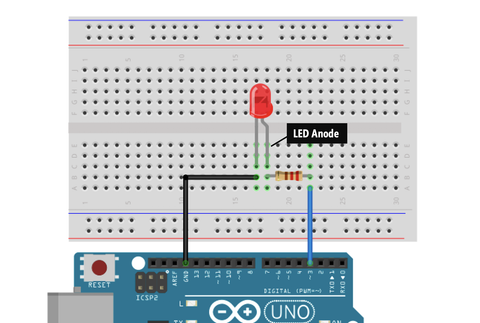# LED Interfacing with Arduino

Today we will learn about LED interfacing with Arduino. Also, what all we require and how can we do it easily.

In this blog, we will see how to interface an LED with Arduino.

## How to interface LED with an Arduino:

For interfacing an LED with Arduino, we need the above-mentioned components and connections have to be made as per the circuit diagram below.

## What is an LED?

LED stands for Light-emitting diode. An LED is a device that produces light whenever current is passed through it.

In this blog, we will blink an LED using Arduino.

## Pinout of LED:## Connection of LED with Arduino:GPIO 3 of Arduino Anode pin of LED via 220ohm resistor GND pin of Arduino Cathode pin of the LED

Make the connections as per the circuit diagram shown in the above image. Next, we have to program our Arduino. For programming our Arduino to blink an LED, we need Arduino IDE to be installed on our PC. You can download and install Arduino IDE suitable for your computer OS from arduino.cc

Once, the Arduino IDE is installed on your PC, we have to write a code to blink an LED in the interval of one second. Below is the code for blinking LED using Arduino.

## Code:

#define led_pin 3

void setup()

{

pinMode(led_pin, OUTPUT);

}

void loop()

{

digitalWrite(led_pin, HIGH);

delay(1000);

digitalWrite(led_pin, LOW);

delay(1000);

}

## Explanation:

#define led_pin 3

#define led_pin 3 : First, we have declared a pin name and pin number to which we are going to connect the LED using #define preprocessor constant. We are assigning GPIO 3 pin of Arduino to connect LED and given a name to that pin as “led_pin”.

void setup()

{

pinMode(led_pin, OUTPUT);

}

void setup() : This function executes only once in our program. In this function, we have called a function called “pinMode(led_pin, OUTPUT);”. The pinMode function is used to tell Arduino, that the connected peripheral is either input or output peripheral. In this function, we are passing the “led_pin” as pin name and “OUTPUT” as type.

void loop()

{

digitalWrite(led_pin, HIGH);

delay(1000);

digitalWrite(led_pin, LOW);

delay(1000);

}

void loop() : This function executes infinitely in our program. In this function, we have called a function “digitalWrite();” in this function, we pass two arguments. Such as the pin name to which the LED is connected and the state of the pin. “digitalWrite(led_pin, HIGH);” makes the led_pin as HIGH means provides 5V at GPIO pin 3. “digitalWrite(led_pin, LOW);” makes the voltage on the GPIO pin 3 to 0V. In between these two functions, we have used a “delay();” function is used to pause the microcontroller for some period of time during the execution. In this program, we are passing “1000” as an argument to the delay(); function. Which gives a delay of 1000 milliseconds. i.e., 1 second.

## Conclusion:

In this blog, we have successfully made a circuit that blinks an LED in an interval of one second using Arduino.

### 1. What is interfacing LED with Arduino?

Interfacing LED with Arduino means, connecting the LED to a GPIO pin of Arduino and controlling it by writing a program.

### 2. Can LED be connected directly to Arduino?

No, we should not connect LED directly to Arduino. As the GPIO pins of Arduino provide 5V on a HIGH state, due to overvoltage, the LED might get damaged. A resistor in series with the LED pin has to be used to avoid over-voltage damage.

### 3. How can you make an LED blink using Arduino Uno?

An LED can be made to blink at an interval of a given time. The code in the above blog can be used to blink an LED using Arduino UNO.

### 4. Do LEDs need resistors on Arduino?

Yes, LEDs need resistors while using with Arduino to avoid over-voltage damage.

## Related Blogs

### 1. What is interfacing LED with Arduino?

Interfacing LED with Arduino means, connecting the LED to a GPIO pin of Arduino and controlling it by writing a program.

### 2. Can LED be connected directly to Arduino?

No, we should not connect LED directly to Arduino. As the GPIO pins of Arduino provide 5V on a HIGH state, due to overvoltage, the LED might get damaged. A resistor in series with the LED pin has to be used to avoid over-voltage damage.

### 3. How can you make an LED blink using Arduino Uno?

An LED can be made to blink at an interval of a given time. The code in the above blog can be used to blink an LED using Arduino UNO.

### 4. Do LEDs need resistors on Arduino?

Yes, LEDs need resistors while using with Arduino to avoid over-voltage damage.